# Function transformations

Function transformations describe how a function can shift, reflect, stretch, and compress. Generally, all transformations can be modeled by the expression:

af(b(x+c))+d

Replacing a, b, c, or d will result in a transformation of that function.

### horizontal and vertical shifts

These shifts occur when the entire function moves vertically or horizontally. They are one of the most basic function transformations. In the general form of function transformations, they are represented by the letters c and d.

Horizontal shifts correspond to the letter c in the general expression. If c is negative, the function will shift right by c units. If c is positive, the function will shift to the left by c units.

You may intuitively think that a positive value should result in a shift in the positive direction, but for horizontal shifts, that is not the case. Just remember that to move in the +x direction, c needs to be negative.

Example

 f(x) = x2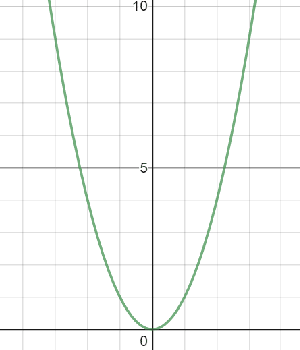f(x) = (x + 3)2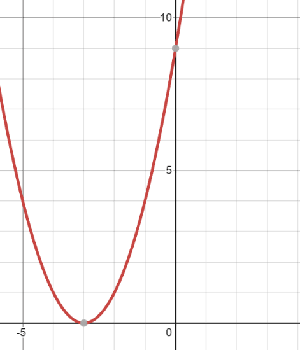Notice that for horizontal shifts, the 3 was not placed outside of x2. For horizontal shifts, you need to add c every time x shows up in the equation.

Vertical shifts correspond to the letter d in the general expression. If d is positive, the function will shift up by d units. If d is negative, the function will shift down by d units.

Unlike horizontal shifts, you do not need to add d every time x shows up in the equation. To vertically shift a function, simply add d onto the end of the function.

Example

 f(x) = x2f(x) = x2 + 3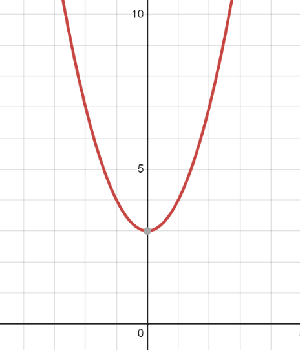### Vertical stretches, compressions, and reflections

These transformations correspond to the letter a in the general expression.

When |a| is greater than 1 a vertical stretch occurs. If the graph were a piece of stretchy fabric, imagine pulling the top and bottom ends of the graph further apart. This is what a vertical stretch looks like.

When we describe a function's vertical stretch, we say that the function is vertically stretched by a factor of |a|.

Example

 f(x) = x2f(x) = 3x2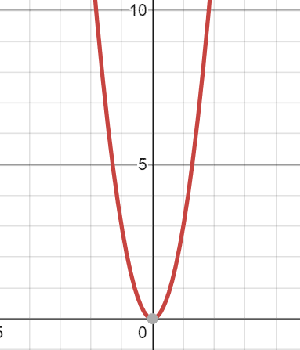When |a| is greater than 0 but less than 1, a vertical compression occurs. Imagine pushing the top and bottom ends of the graph together. This is what a vertical compression looks like.

When we describe a function's vertical compression, we say that the function is vertically compressed by a factor of |a|.

Example

 f(x) = x2f(x) =x2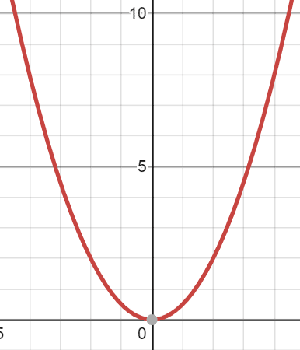If a is negative, the graph is reflected vertically across the x-axis. This transformation is called a reflection.

Example

 f(x) = x2f(x) = -x2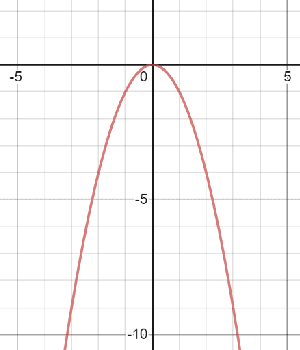To create a vertical stretch, compression, or reflection, the entire function needs to be multiplied by a.

### Horizontal stretches, compressions, and reflections

These occur when b is replaced by any real number.

When |b| is greater than 1, a horizontal compression occurs. A horizontal compression looks similar to a vertical stretch.

When we describe a function's horizontal compression, we say that the function is horizontally compressed by a factor of ||.

Example

 f(x) = x2f(3x) = (3x)2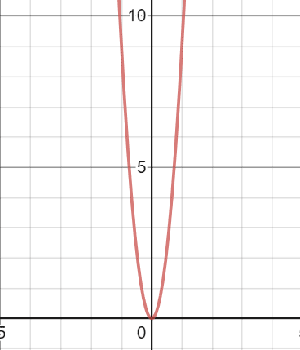When |b| is greater than 0 but less than 1, a horizontal stretch occurs. A horizontal stretch looks similar to a vertical compression.

When we describe a function's horizontal stretch, we say that the function is horizontally stretched by a factor of ||.

Example

 f(x) = x2f(x) = (x)2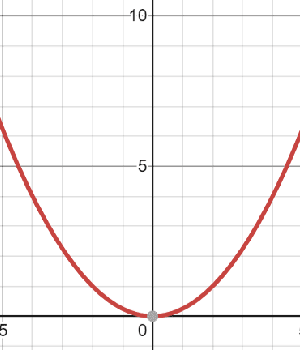If b is negative, the graph is reflected horizontally across the y-axis. f(x) = x2 is an even function, so reflecting it over the y-axis does not change its graph. Let's look at f(x) = x3 instead.

Example

 f(x) = x3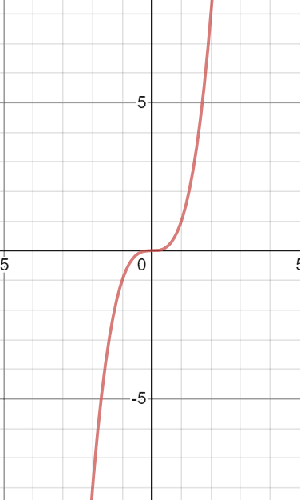f(x) = (-x)3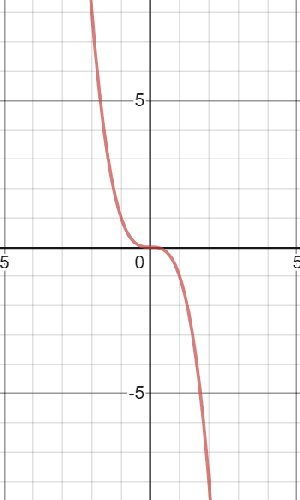To create a horizontal stretch, compression, or reflection, you need to multiply by b every time x shows up in the function.

### handling multiple transformations

You may be asked to write or graph a function with multiple transformations. In this case, you follow something similar to order of operations:

1. Parentheses (horizontal shifts, c)
2. Multiplication (horizontal stretches, compressions, and reflections, b)
3. Multiplication (vertical stretches, compressions, and reflections, a)
4. Addition and subtraction (vertical shifts, d)

ExampleStart with the parent function:First, shift the graph horizontally 4 units to the right.

Second, compress the graph horizontally by a factor of.

Third, vertically stretch the graph by a factor of 2.

Fourth, reflect the graph across the x-axis.

Lastly, vertically shift the graph up by 1 unit.